Beams Index

Continuous Beams

Introduction

Built -in (Encastre) beams are fixed at both ends.   Continuous beams, which are beams with more than two supports and covering more than one span, are not statically determinate using the static equilibrium laws.

Nomenclature

e = strain
σ = stress (N/m2)
E = Young's Modulus = σ /e (N/m2)
y = distance of surface from neutral surface (m).
R = Radius of neutral axis (m).
I = Moment of Inertia (m4 - more normally cm4)
Z = section modulus = I/y max(m3 - more normally cm3)
M = Moment (Nm)
w = Distributed load on beam (kg/m) or (N/m as force units)
W = total load on beam (kg ) or (N as force units)
F= Concentrated force on beam (N)
L = length of beam (m)
x = distance along beam (m)

Built in beams

A built in beam is normally considered to be horizontal with both ends built-in at the same level and with zero slope at both ends.   A loaded built in beam has a moment at both ends and normally the maximum moments at at one or both of the two end joints.

A built in beam is generally much stronger than a simply supported beam of the same geometry.  The bending moment reduces along the beam and changes sign at points of contraflexure between the supports and the load.   A typical built-in beam is shown below.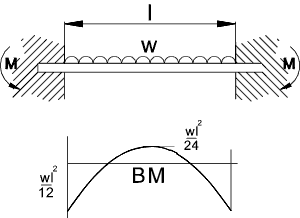It is not normally possible to determine the bending moments and the resulting stress using static equilibrium.   Deflection calculations are often used to enable the moments to be determined.

Repeating section from Beam Theory

It has been proved ref Shear Bending that dM/dx = S and dS/dx = -w = d2M /dx

Where S = the shear force M is the moment and w is the distributed load /unit length of beam. Therefore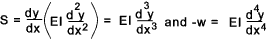Using the above beam as an example...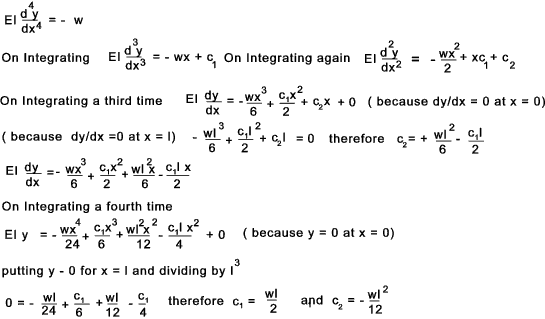Using the above equations the bending moment, shear force, deflection, slope can be determined at any point along the beam.

M = EI d 2y/dx 2 = w(- 6x2+6lx -l2)/12

at x = 0 & l then M = -wl2 /12 and at x = l/2 then M = wl2 /24

S = EI d 3y/dx 3 = w(l/2 - x)
at x = 0 then S = w.l/2 at x = l then S = -w.l/2

Continuous Beams

This type of beam is normally considered using the Clapeyron's Theorem ( Three Moments theorem)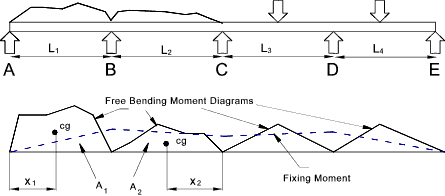The three moments theorem identifies the relationship between the bending moments found at three consecutive supports in a continuous beam.  This is achieved by evaluating the slope of of the beam at the end where the two spans join.  The slopes are expressed in terms of the three moments and the supported loads which are then equated and the resulting equations solved.

This relationship for spans with supports at the same height and with spans of constant section results in the following expression.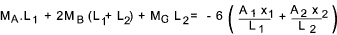If the beams has a different section for each span then the more general expression applies as shown below.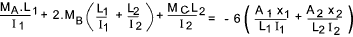Example Areas and x 1 value calculations.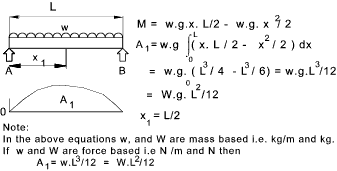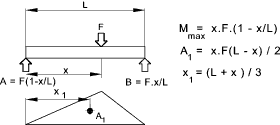Examples using theorem.

Example 1)

This simple example is a two span continuous beam with the ends simple supported,therefore with no moments at the end support points..

1) Bending Moments.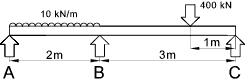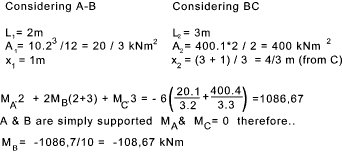2) Shear Forces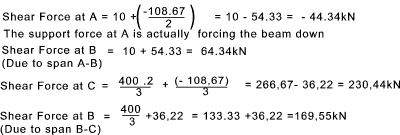3) Diagrams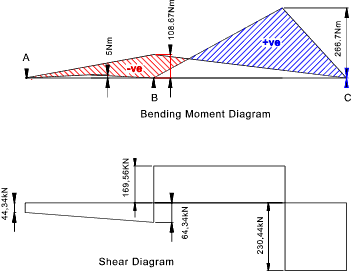Example 2)

This simple example is a three span continuous beam with the ends simple supported,therefore with no moments at the end support points..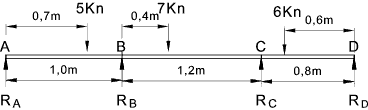The values of A1,x1,A2, and x2 are calculated using the methods above..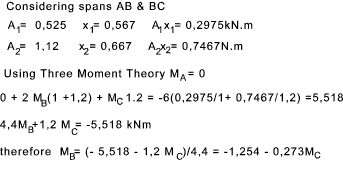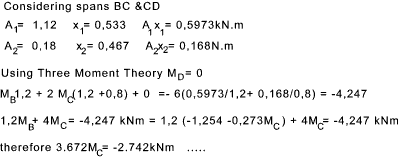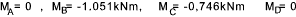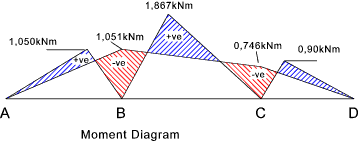Sites Providing Relevant Information Three Moment equation for continous beam analysis...Very advanced paper download Mitcalc...Excel based software including coded beam calculations Granta -Solutions to Standard Problems...Very accessible notes for beams and sections Chaapter 6 Moment Distribution...Chapter of Book inlcuding detailed information
Beams Index